Next: Spherical harmonics Up: Useful mathematics Previous: Elliptic expansions

# Matrix eigenvalue theory

Suppose that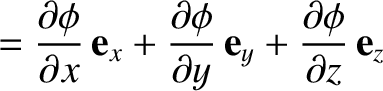is a real symmetric square matrix of dimension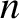. If follows that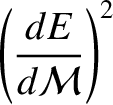and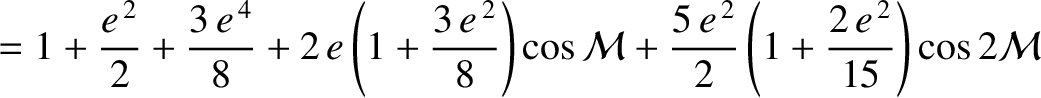, where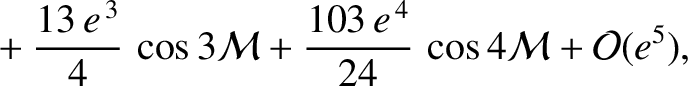denotes a complex conjugate, and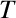denotes a transpose. Consider the matrix equation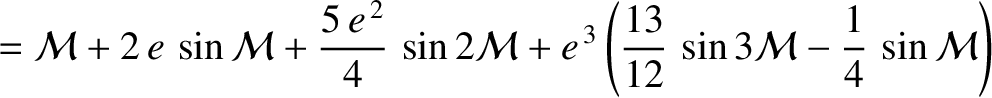(A.144)

Any column vector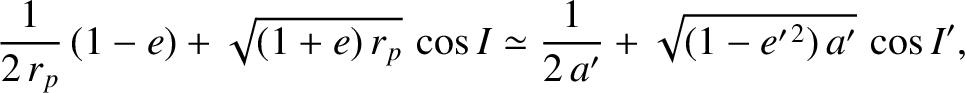which satisfies this equation is called an eigenvector of. Likewise, the associated number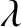is called an eigenvalue of(Gradshteyn and Ryzhik 1980c). Let us investigate the properties of the eigenvectors and eigenvalues of a real symmetric matrix.

Equation (A.144) can be rearranged to give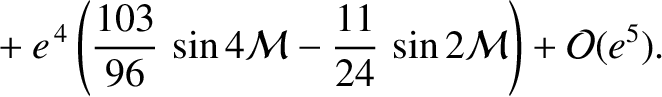(A.145)

where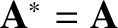is the unit matrix. The preceding matrix equation is essentially a set ofhomogeneous simultaneous algebraic equations for thecomponents of. A well-known property of such a set of equations is that it only has a nontrivial solution when the determinant of the associated matrix is set to zero (Gradshteyn and Ryzhik 1980c). Hence, a necessary condition for the preceding set of equations to have a nontrivial solution is that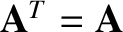(A.146)

where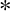denotes a determinant. This formula is essentially anth-order polynomial equation for. We know that such an equation has(possibly complex) roots. Hence, we conclude that there areeigenvalues, andassociated eigenvectors, of the-dimensional matrix.

Let us now demonstrate that theeigenvalues and eigenvectors of the real symmetric matrixare all real. We have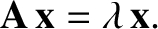(A.147)

and, taking the transpose and complex conjugate,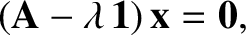(A.148)

where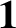andare the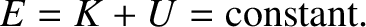th eigenvector and eigenvalue of, respectively. Left multiplying Equation (A.147) by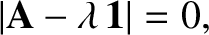, we obtain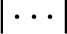(A.149)

Likewise, right multiplying Equation (A.148) by, we get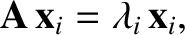(A.150)

The difference of the previous two equations yields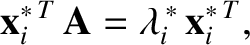(A.151)

It follows that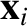, because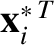(which is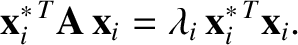in vector notation) is real and positive definite. Hence,is real. It immediately follows thatis real.

Next, let us show that two eigenvectors corresponding to two different eigenvalues are mutually orthogonal. Let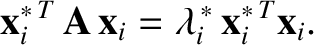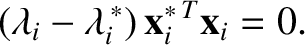(A.152) and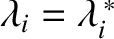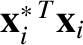(A.153)

where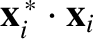. Taking the transpose of the first equation and right multiplying by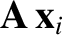, and left multiplying the second equation by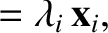, we obtain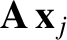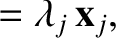(A.154) and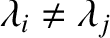(A.155)

Taking the difference of the preceding two equations, we get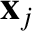(A.156)

Because, by hypothesis,, it follows that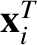. In vector notation, this is the same as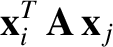. Hence, the eigenvectorsandare mutually orthogonal.

Suppose that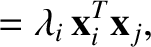. In this case, we cannot conclude thatby the preceding argument. However, it is easily seen that any linear combination ofandis an eigenvector ofwith eigenvalue. Hence, it is possible to define two new eigenvectors of, with the eigenvalue, which are mutually orthogonal. For instance,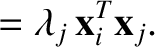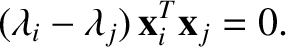(A.157) and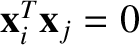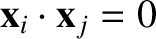(A.158)

It should be clear that this argument can be generalized to deal with any number of eigenvalues that take the same value.

In conclusion, a real symmetric-dimensional matrix possessesreal eigenvalues, withassociated real eigenvectors, which are, or can be chosen to be, mutually orthogonal.Next: Spherical harmonics Up: Useful mathematics Previous: Elliptic expansions
Richard Fitzpatrick 2016-03-31